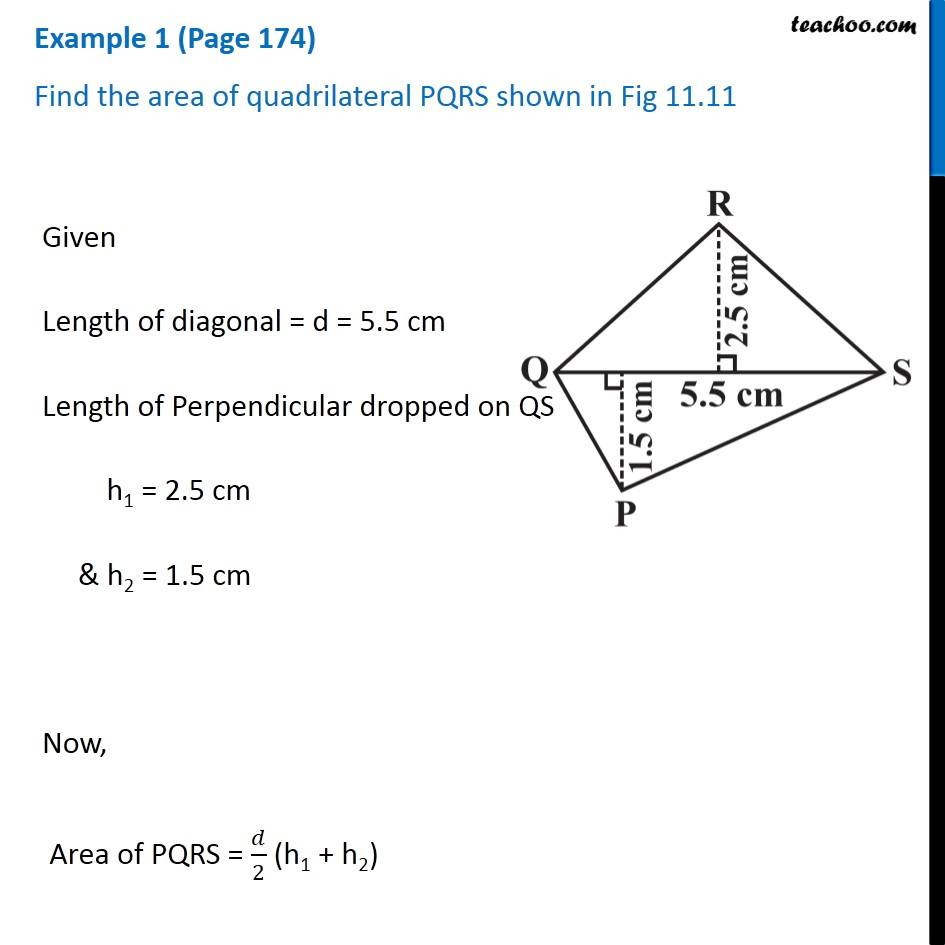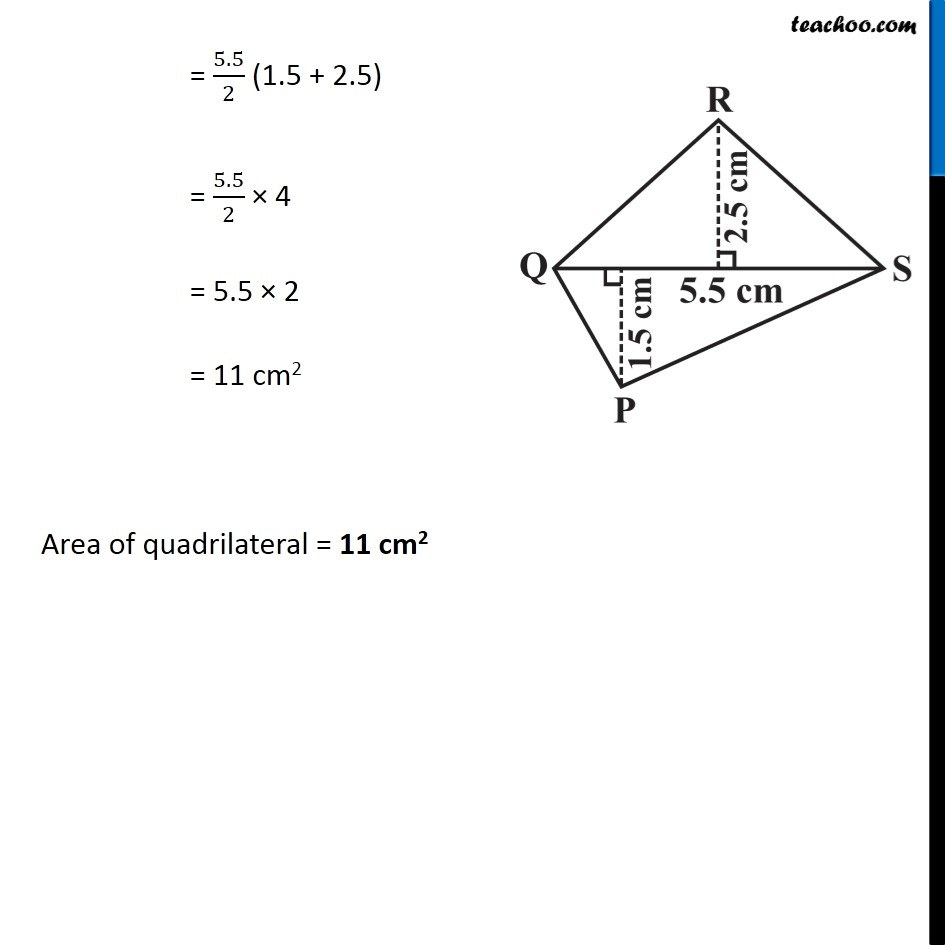Examples

Chapter 11 Class 8 Mensuration
Serial order wiseGet live Maths 1-on-1 Classs - Class 6 to 12

### Transcript

Example 1 (Page 174) Find the area of quadrilateral PQRS shown in Fig 11.11 Given Length of diagonal = d = 5.5 cm Length of Perpendicular dropped on QS h1 = 2.5 cm & h2 = 1.5 cm Now, Area of PQRS = 𝑑/2 (h1 + h2) = 5.5/2 (1.5 + 2.5) = 5.5/2 × 4 = 5.5 × 2 = 11 cm2 Area of quadrilateral = 11 cm2